• 详细来讲讲梯度下降算法的原理，感受数学和程序的魅力吧！！
博文目录1. 概述2. 梯度下降算法2.1 场景假设2.2 梯度下降2.2.1 微分2.2.2 梯度2.3 数学解释2.3.1 α2.3.2 梯度要乘以一个负号3. 实例3.1 单变量函数的梯度下降3.2 多变量函数的梯度下降4. 代码实现4. 1 场景分析4. 2 代码5. 小结
1. 概述
本文将从一个下山的场景开始，先提出梯度下降算法的基本思想，进而从数学上解释梯度下降算法的原理，解释为什么要用梯度，最后实现一个简单的梯度下降算法的实例！
2. 梯度下降算法
2.1 场景假设
梯度下降法的基本思想可以类比为一个下山的过程。
假设这样一个场景：一个人被困在山上，需要从山上下来(找到山的最低点，也就是山谷)。但此时山上的浓雾很大，导致可视度很低；因此，下山的路径就无法确定，必须利用自己周围的信息一步一步地找到下山的路。这个时候，便可利用梯度下降算法来帮助自己下山。怎么做呢，首先以他当前的所处的位置为基准，寻找这个位置最陡峭的地方，然后朝着下降方向走一步，然后又继续以当前位置为基准，再找最陡峭的地方，再走直到最后到达最低处；同理上山也是如此，只是这时候就变成梯度上升算法了2.2 梯度下降
梯度下降的基本过程就和下山的场景很类似。
首先，我们有一个可微分的函数。这个函数就代表着一座山。我们的目标就是找到这个函数的最小值，也就是山底。根据之前的场景假设，最快的下山的方式就是找到当前位置最陡峭的方向，然后沿着此方向向下走，对应到函数中，就是找到给定点的梯度 ，然后朝着梯度相反的方向，就能让函数值下降的最快！因为梯度的方向就是函数之变化最快的方向(在后面会详细解释)
所以，我们重复利用这个方法，反复求取梯度，最后就能到达局部的最小值，这就类似于我们下山的过程。而求取梯度就确定了最陡峭的方向，也就是场景中测量方向的手段。那么为什么梯度的方向就是最陡峭的方向呢？接下来，我们从微分开始讲起：
2.2.1 微分
看待微分的意义，可以有不同的角度，最常用的两种是：

函数图像中，某点的切线的斜率
函数的变化率
几个微分的例子：

1.单变量的微分，函数只有一个变量时
$\frac{d(x^2)}{dx}=2x$
$\frac{d(-2y^5)}{dy}=-10y^4$
$\frac{d(5-\theta )^2}{d\theta}=-2(5-\theta)$
2.多变量的微分，当函数有多个变量的时候，即分别对每个变量进行求微分
$\frac{\partial}{\partial x}(x^2y^2) = 2xy^2$
$\frac{\partial}{\partial y}(-2y^5+z^2) = -10y^4$
$\frac{\partial}{\partial \theta_{2}}(5\theta_{1} + 2\theta_{2} - 12\theta_{3}) = 2$
$\frac{\partial}{\partial \theta_{2}}(0.55 - (5\theta_{1} + 2\theta_{2} - 12\theta_{3})) = -2$
2.2.2 梯度
梯度实际上就是多变量微分的一般化。
下面这个例子：
$J(\Theta ) = 0.55 - (5\theta_{1} + 2\theta_{2} - 12\theta_{3})$
$\triangledown J(\Theta ) = \left < \frac{\partial J}{\partial \theta_{1}}, \frac{\partial J}{\partial \theta_{2}},\frac{\partial J}{\partial \theta_{3}} \right > =(-5,-2,12)$
我们可以看到，梯度就是分别对每个变量进行微分，然后用逗号分割开，梯度是用<>包括起来，说明梯度其实一个向量。
梯度是微积分中一个很重要的概念，之前提到过梯度的意义

在单变量的函数中，梯度其实就是函数的微分，代表着函数在某个给定点的切线的斜率
在多变量函数中，梯度是一个向量，向量有方向，梯度的方向就指出了函数在给定点的上升最快的方向

这也就说明了为什么我们需要千方百计的求取梯度！我们需要到达山底，就需要在每一步观测到此时最陡峭的地方，梯度就恰巧告诉了我们这个方向。梯度的方向是函数在给定点上升最快的方向，那么梯度的反方向就是函数在给定点下降最快的方向，这正是我们所需要的。所以我们只要沿着梯度的方向一直走，就能走到局部的最低点！
2.3 数学解释
首先给出数学公式：
${\color{Red} \Theta^1} = {\color{Blue} \Theta^0} + {\color{Green} \alpha} {\color{Purple} \triangledown J(\Theta)}\rightarrow evaluated at \Theta^0$
此公式的意义是：J是关于Θ的一个函数，我们当前所处的位置为Θ0点，要从这个点走到J的最小值点，也就是山底。首先我们先确定前进的方向，也就是梯度的反向，然后走一段距离的步长，也就是α，走完这个段步长，就到达了Θ1这个点！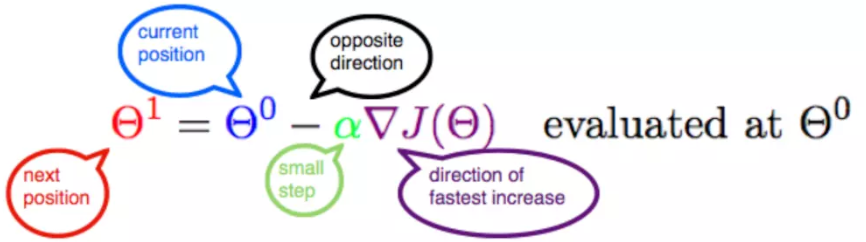2.3.1 α
α在梯度下降算法中被称作为学习率或者步长，意味着我们可以通过α来控制每一步走的距离，以保证不要步子跨的太大扯着蛋，哈哈，其实就是不要走太快，错过了最低点。同时也要保证不要走的太慢，导致太阳下山了，还没有走到山下。所以α的选择在梯度下降法中往往是很重要的！α不能太大也不能太小，太小的话，可能导致迟迟走不到最低点，太大的话，会导致错过最低点！
2.3.2 梯度要乘以一个负号
梯度前加一个负号，就意味着朝着梯度相反的方向前进！我们在前文提到，梯度的方向实际就是函数在此点上升最快的方向！而我们需要朝着下降最快的方向走，自然就是负的梯度的方向，所以此处需要加上负号；那么如果时上坡，也就是梯度上升算法，当然就不需要添加负号了。
3. 实例
我们已经基本了解了梯度下降算法的计算过程，那么我们就来看几个梯度下降算法的小实例，首先从单变量的函数开始，然后介绍多变量的函数。
3.1 单变量函数的梯度下降
我们假设有一个单变量的函数
$J(\theta) = \theta^2$
函数的微分，直接求导就可以得到
$J'(\theta) = 2\theta$
初始化，也就是起点，起点可以随意的设置，这里设置为1
$\theta^0 = 1$
学习率也可以随意的设置，这里设置为0.4
$\alpha = 0.4$
根据梯度下降的计算公式
${\color{Red} \Theta^1} = {\color{Blue} \Theta^0} + {\color{Green} \alpha} {\color{Purple} \triangledown J(\Theta)}\rightarrow evaluated at \Theta^0$
我们开始进行梯度下降的迭代计算过程：
$\theta^0 = 1$
$\theta^1 = \theta^0 - \alpha*J'(\theta^0)=1 - 0.4*2 = 0.2$
$\theta^2 = \theta^1 - \alpha*J'(\theta^1)= 0.2 - 0.4*0.4=0.04$
$\theta^3 = 0.008$
$\theta^4 = 0.0016$
如图，经过四次的运算，也就是走了四步，基本就抵达了函数的最低点，也就是山底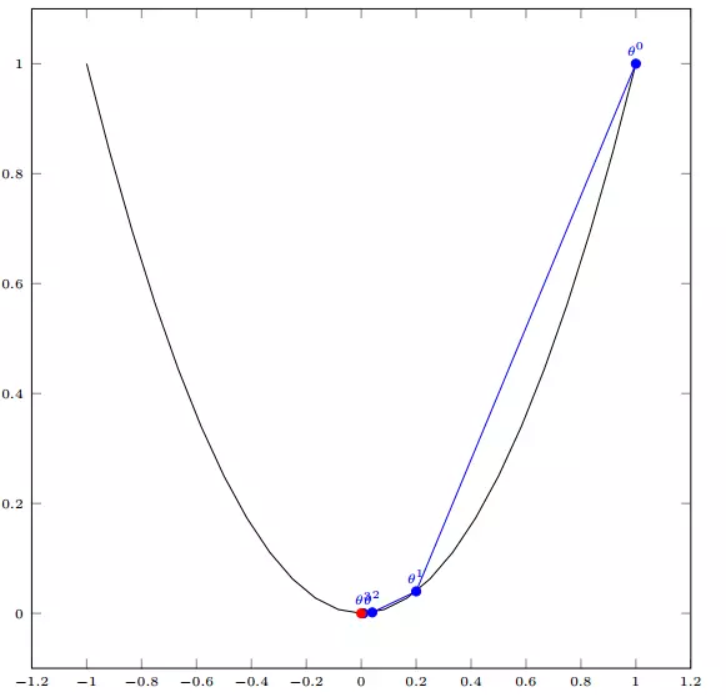3.2 多变量函数的梯度下降
我们假设有一个目标函数
$J(\Theta) = \theta_{1}^2 + \theta_{2}^2$
现在要通过梯度下降法计算这个函数的最小值。我们通过观察就能发现最小值其实就是 (0，0)点。但是接下来，我们会从梯度下降算法开始一步步计算到这个最小值！
我们假设初始的起点为：
$\Theta^0 = (1, 3)$
初始的学习率为：
$\alpha = 0.1$
函数的梯度为：
$\triangledown J(\Theta ) = \left < 2\theta_{1},2\theta_{2} \right >$
进行多次迭代：
$\Theta^0 = (1, 3)$
$\Theta^1 = \Theta^0 - \alpha\triangledown J(\Theta ) = (1,3) - 0.1*(2, 6)=(0.8, 2.4)$
$\Theta^2 = (0.8, 2.4) - 0.1*(1.6, 4.8)=(0.64, 1.92)$
$\Theta^3 =(0.5124, 1.536)$
$\Theta^4 =(0.4096, 1.228800000000001)$
$\vdots$
$\Theta^{10} =(0.1073741824000003, 0.32212254720000005)$
$\vdots$
$\Theta^{50} =(1.141798154164342e^{-05}, 3.42539442494306e^{-05})$
$\vdots$
$\Theta^{100} =(1.6296287810675902e^{-10}, 4.8888886343202771e^{-10})$
我们发现，已经基本靠近函数的最小值点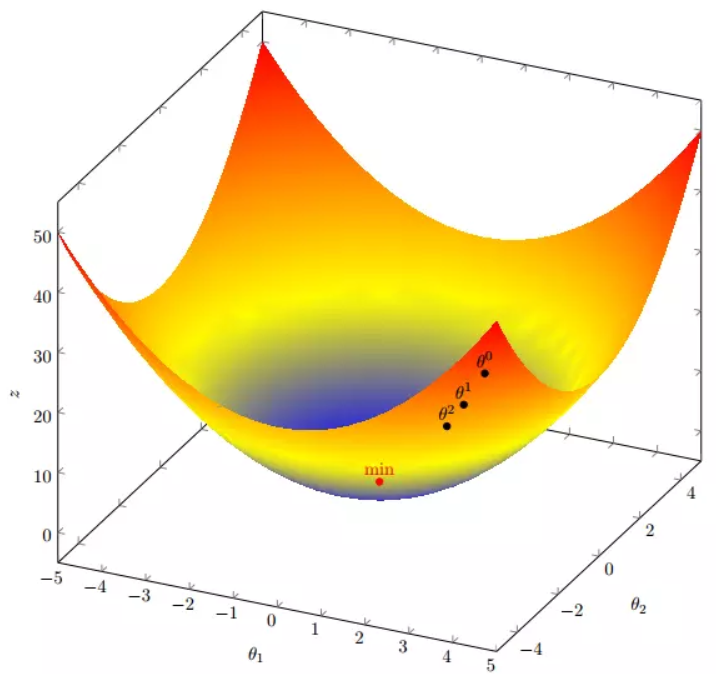4. 代码实现
4. 1 场景分析
下面我们将用python实现一个简单的梯度下降算法。场景是一个简单的线性回归的例子：假设现在我们有一系列的点，如下图所示：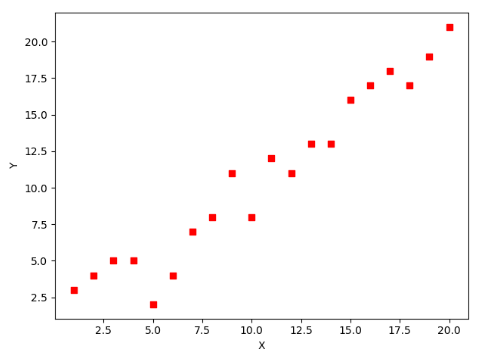我们将用梯度下降法来拟合出这条直线！
首先，我们需要定义一个代价函数，在此我们选用均方误差代价函数（也称平方误差代价函数）
$J(\Theta) = \frac{1}{2m}\sum_{i=1}^{m}(h_{\theta}(x^{(i)})-y^{(i)})^2$
此公式中

m是数据集中数据点的个数，也就是样本数
½是一个常量，这样是为了在求梯度的时候，二次方乘下来的2就和这里的½抵消了，自然就没有多余的常数系数，方便后续的计算，同时对结果不会有影响
y 是数据集中每个点的真实y坐标的值，也就是类标签
h 是我们的预测函数（假设函数），根据每一个输入x，根据Θ 计算得到预测的y值，即

$h_{\Theta}(x^{(i)}) = \Theta_{0} + \Theta_{1}x_{1}^{(i)}$
我们可以根据代价函数看到，代价函数中的变量有两个，所以是一个多变量的梯度下降问题，求解出代价函数的梯度，也就是分别对两个变量进行微分
$\triangledown J(\Theta ) = \left < \frac{\delta J}{\delta \Theta_{0}}, \frac{\delta J}{\delta \Theta_{1}} \right >$
$\frac{\delta J}{\delta \Theta_{0}} = \frac{1}{m}\sum_{i=1}^{m}(h_{\Theta}(x^{(i)})-y^{(i)})$
$\frac{\delta J}{\delta \Theta_{1}} = \frac{1}{m}\sum_{i=1}^{m}(h_{\Theta}(x^{(i)})-y^{(i)})x_{1}^{(i)}$
明确了代价函数和梯度，以及预测的函数形式。我们就可以开始编写代码了。但在这之前，需要说明一点，就是为了方便代码的编写，我们会将所有的公式都转换为矩阵的形式，python中计算矩阵是非常方便的，同时代码也会变得非常的简洁。
为了转换为矩阵的计算，我们观察到预测函数的形式
$h_{\Theta}(x^{(i)}) = \Theta_{0} + \Theta_{1}x^{(i)}$
我们有两个变量，为了对这个公式进行矩阵化，我们可以给每一个点x增加一维，这一维的值固定为1，这一维将会乘到Θ0上。这样就方便我们统一矩阵化的计算
$(x_{1}^{(i)},y^{(i)})\rightarrow (x_{0}^{(i)},x_{1}^{(i)},y^{(i)}) with x_{0}^{(i)} = 1 \forall _{i}$
然后我们将代价函数和梯度转化为矩阵向量相乘的形式
$J(\Theta) = \frac{1}{2m}(X\Theta - \vec{y})^{T}(X\Theta - \vec{y})$
$\triangledown J(\Theta) = \frac{1}{m}X^{T}(X\Theta - \vec{y}))$
4. 2 代码
首先，我们需要定义数据集和学习率
#!/usr/bin/env python3
# -*- coding: utf-8 -*-
# @Time    : 2019/1/21 21:06
# @Author  : Arrow and Bullet
# @Software: PyCharm
# @Blog    ：https://blog.csdn.net/qq_41800366

from numpy import *

# 数据集大小 即20个数据点
m = 20
# x的坐标以及对应的矩阵
X0 = ones((m, 1))  # 生成一个m行1列的向量，也就是x0，全是1
X1 = arange(1, m+1).reshape(m, 1)  # 生成一个m行1列的向量，也就是x1，从1到m
X = hstack((X0, X1))  # 按照列堆叠形成数组，其实就是样本数据
# 对应的y坐标
y = np.array([
3, 4, 5, 5, 2, 4, 7, 8, 11, 8, 12,
11, 13, 13, 16, 17, 18, 17, 19, 21
]).reshape(m, 1)
# 学习率
alpha = 0.01

接下来我们以矩阵向量的形式定义代价函数和代价函数的梯度
# 定义代价函数
def cost_function(theta, X, Y):
diff = dot(X, theta) - Y  # dot() 数组需要像矩阵那样相乘，就需要用到dot()
return (1/(2*m)) * dot(diff.transpose(), diff)

# 定义代价函数对应的梯度函数
diff = dot(X, theta) - Y
return (1/m) * dot(X.transpose(), diff)

最后就是算法的核心部分，梯度下降迭代计算
# 梯度下降迭代
theta = array([1, 1]).reshape(2, 1)
theta = theta - alpha * gradient
return theta

print('optimal:', optimal)
print('cost function:', cost_function(optimal, X, Y))

当梯度小于1e-5时，说明已经进入了比较平滑的状态，类似于山谷的状态，这时候再继续迭代效果也不大了，所以这个时候可以退出循环！
运行代码，计算得到的结果如下：
print('optimal:', optimal)  # 结果 [[0.51583286][0.96992163]]
print('cost function:', cost_function(optimal, X, Y))  # 1.014962406233101

通过matplotlib画出图像，
# 根据数据画出对应的图像
def plot(X, Y, theta):
import matplotlib.pyplot as plt
ax = plt.subplot(111)  # 这是我改的
ax.scatter(X, Y, s=30, c="red", marker="s")
plt.xlabel("X")
plt.ylabel("Y")
x = arange(0, 21, 0.2)  # x的范围
y = theta + theta*x
ax.plot(x, y)
plt.show()

plot(X1, Y, optimal)

所拟合出的直线如下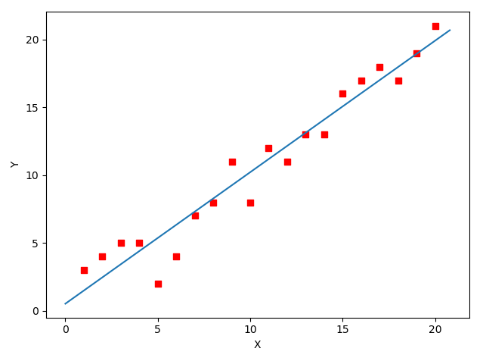全部代码如下，大家有兴趣的可以复制下来跑一下看一下结果：
#!/usr/bin/env python3
# -*- coding: utf-8 -*-
# @Time    : 2019/1/21 21:06
# @Author  : Arrow and Bullet
# @Software: PyCharm
# @Blog    ：https://blog.csdn.net/qq_41800366

from numpy import *

# 数据集大小 即20个数据点
m = 20
# x的坐标以及对应的矩阵
X0 = ones((m, 1))  # 生成一个m行1列的向量，也就是x0，全是1
X1 = arange(1, m+1).reshape(m, 1)  # 生成一个m行1列的向量，也就是x1，从1到m
X = hstack((X0, X1))  # 按照列堆叠形成数组，其实就是样本数据
# 对应的y坐标
Y = array([
3, 4, 5, 5, 2, 4, 7, 8, 11, 8, 12,
11, 13, 13, 16, 17, 18, 17, 19, 21
]).reshape(m, 1)
# 学习率
alpha = 0.01

# 定义代价函数
def cost_function(theta, X, Y):
diff = dot(X, theta) - Y  # dot() 数组需要像矩阵那样相乘，就需要用到dot()
return (1/(2*m)) * dot(diff.transpose(), diff)

# 定义代价函数对应的梯度函数
diff = dot(X, theta) - Y
return (1/m) * dot(X.transpose(), diff)

# 梯度下降迭代
theta = array([1, 1]).reshape(2, 1)
theta = theta - alpha * gradient
return theta

print('optimal:', optimal)
print('cost function:', cost_function(optimal, X, Y))

# 根据数据画出对应的图像
def plot(X, Y, theta):
import matplotlib.pyplot as plt
ax = plt.subplot(111)  # 这是我改的
ax.scatter(X, Y, s=30, c="red", marker="s")
plt.xlabel("X")
plt.ylabel("Y")
x = arange(0, 21, 0.2)  # x的范围
y = theta + theta*x
ax.plot(x, y)
plt.show()

plot(X1, Y, optimal)

5. 小结
至此，就基本介绍完了梯度下降法的基本思想和算法流程，并且用python实现了一个简单的梯度下降算法拟合直线的案例！
最后，我们回到文章开头所提出的场景假设:
这个下山的人实际上就代表了反向传播算法，下山的路径其实就代表着算法中一直在寻找的参数Θ，山上当前点的最陡峭的方向实际上就是代价函数在这一点的梯度方向，场景中观测最陡峭方向所用的工具就是微分 。在下一次观测之前的时间就是有我们算法中的学习率α所定义的。
可以看到场景假设和梯度下降算法很好的完成了对应！
本文部分内容来自一位前辈，非常感谢分享！谢谢！


展开全文梯度下降
• 在本篇文章里小编给大家整理的是一篇关于python实现梯度下降算法实例详解内容，需要的朋友们可以参考下。
• 梯度下降算法的正确步骤Title: What is the Gradient Descent Algorithm and its working. 标题:什么是梯度下降算法及其工作原理。 Gradient descent is a type of machine learning algorithm that helps us in ...
梯度下降算法的正确步骤Title: What is the Gradient Descent Algorithm and its working. 标题：什么是梯度下降算法及其工作原理。
Gradient descent is a type of machine learning algorithm that helps us in optimizing neural networks and many other algorithms. This article ventures into how this algorithm actually works, its types, and its significance in the real world. 梯度下降是一种机器学习算法，可帮助我们优化神经网络和许多其他算法。 本文探讨了该算法的实际工作原理，类型及其在现实世界中的重要性。
简介 (A Brief Introduction)
Gradient descent is one of the most popular algorithms to perform optimization and by far the most common way to optimize neural networks. At the same time, every state-of-the-art Deep Learning library contains implementations of various algorithms to optimize gradient descent (e.g. lasagne’s, caffe’s, and keras’ documentation). 梯度下降是执行优化的最流行算法之一，也是迄今为止最优化神经网络的最常用方法。 同时，每个最新的深度学习库都包含各种算法的实现，以优化梯度下降(例如，千层面，咖啡和keras的文档)。
The reason we’re talking about it here is not merely theoretical. Gradient Descent algorithm is much more than it seems to be. It is used time and again by ML practitioners, Data scientists, and students to optimize their models. 我们在这里谈论它的原因不仅仅是理论上的。 梯度下降算法远不止于此。 机器学习从业人员，数据科学家和学生反复使用它来优化模型。
Gradient descent is a way to minimize an objective function parameterized by a model’s parameters by updating the parameters in the opposite direction of the gradient of the objective function w.r.t. to the parameters. The learning rate $alpha$ determines the size of the steps we take to reach a (local) minimum. In other words, we follow the direction of the slope of the surface created by the objective function downhill until we reach a valley. 梯度下降是一种通过在目标函数wrt与参数的梯度相反的方向上更新参数来最小化由模型的参数参数化的目标函数的方法。 学习率$alpha$决定了我们达到(本地)最小值的步骤的大小。 换句话说，我们遵循由下坡的目标函数创建的表面的坡度方向，直到到达山谷。
Now that you’ve gotten a basic insight of the algorithm, let’s dig deep in it in this post. We will define and cover some important aspects like its working, it’s working examples, types and a final conclusion to mould it all. 现在您已经对该算法有了基本的了解，让我们在本文中深入研究它。 我们将定义并涵盖一些重要方面，例如其工作，它的工作示例，类型以及塑造这一切的最终结论。
什么是梯度下降？ (What is exactly Gradient Descent ?)
Answer the question posed by the title of this post directly below this header. This will increase your chances of ranking for the featured snippet on Google for this phrase and provide readers with an immediate answer. Keep the length of this definition — at least in this very basic introduction — between 50 and 60 words. 回答此标题正下方的帖子标题所提出的问题。 这将增加您对该词在Google上的精选摘要进行排名的机会，并为读者提供立即的答案。 至少在本基本介绍中，此定义的长度应保持在50到60个字之间。
After the brief definition, dive further into the concept and add more context and explanation if needed. 在简要定义之后，请进一步深入该概念，并在需要时添加更多上下文和说明。
Gradient descent is an optimization algorithm used to find the values of parameters (coefficients) of a function (f) that minimizes a cost function (cost). 梯度下降是一种优化算法，用于查找使成本函数(cost)最小的函数(f)的参数(系数)的值。
Gradient descent is best used when the parameters cannot be calculated analytically (e.g. using linear algebra) and must be searched for by an optimization algorithm. 当无法解析计算参数(例如使用线性代数)并且必须通过优化算法进行搜索时，最好使用梯度下降。
Gradient descent is a first-order iterative optimization algorithm for finding a local minimum of a differentiable function. To find a local minimum of a function using gradient descent, we take steps proportional to the negative of the gradient (or approximate gradient) of the function at the current point. But if we instead take steps proportional to the positive of the gradient, we approach a local maximum of that function; the procedure is then known as gradient ascent. Gradient descent was originally proposed by Cauchy in 1847. 梯度下降是用于找到可微函数的局部最小值的一阶 迭代 优化 算法 。 要使用梯度下降找到函数的局部最小值，我们采取与该函数在当前点的梯度 (或近似梯度)的负值成比例的步骤。 但是，如果我们改为采取与梯度的正比成比例的步骤，则会逼近该函数的局部最大值 。 该过程称为梯度上升 。 梯度下降最初是由柯西在1847年提出的。
Gradient descent is also known as steepest descent; but gradient descent should not be confused with the method of steepest descent for approximating integrals. 梯度下降也被称为最陡下降 ; 但是，不应将梯度下降与近似积分的最速下降方法混淆。
好的，但是为什么重要呢？ (Okay but why is it Important?)
Provide your readers with a few reasons why they should care about the term or the concept you’re writing about. If this is a consumer-level concept, talk about the implications this could have on their businesses, finances, personal happiness, etc. If you’re writing for an audience of professionals, mention the impact this term or concept has on profit, efficiency, and/or customer satisfaction. To make the most of this section, make sure it includes at least one statistic, quote, or outside reference. 为您的读者提供一些理由，让他们了解自己正在写的术语或概念。 如果这是一个消费者级别的概念，请谈论这可能对他们的业务，财务状况，个人幸福感等产生的影响。如果您是为专业人士而写的，请提及此术语或概念对利润，效率的影响和/或客户满意度。 要充分利用本节的内容，请确保它至少包含一个统计信息，引用或外部参考。
Include at Least One of These Next Three Sections 至少包括以下三个部分中的一个
There are three variants of gradient descent, which differ in how much data we use to compute the gradient of the objective function. Depending on the amount of data, we make a trade-off between the accuracy of the parameter update and the time it takes to perform an update. 梯度下降有三种变体，它们在计算目标函数的梯度时使用多少数据不同。 根据数据量，我们在参数更新的准确性和执行更新所需的时间之间进行权衡。
Batch Gradient Descent: This is a type of gradient descent which processes all the training examples for each iteration of gradient descent. But if the number of training examples is large, then batch gradient descent is computationally very expensive. Hence if the number of training examples is large, then batch gradient descent is not preferred. Instead, we prefer to use stochastic gradient descent or mini-batch gradient descent. 批梯度下降：这是一种梯度下降，它为每次梯度下降迭代处理所有训练示例。 但是，如果训练示例的数量很大，那么批梯度下降在计算上将非常昂贵。 因此，如果训练示例的数量很大，则不优选批量梯度下降。 相反，我们更喜欢使用随机梯度下降或小批量梯度下降。 Stochastic Gradient Descent: This is a type of gradient descent which processes 1 training example per iteration. Hence, the parameters are being updated even after one iteration in which only a single example has been processed. Hence this is quite faster than batch gradient descent. But again, when the number of training examples is large, even then it processes only one example which can be additional overhead for the system as the number of iterations will be quite large. 随机梯度下降：这是一种梯度下降，每次迭代处理1个训练示例。 因此，即使在仅处理了一个示例的一次迭代之后，也要更新参数。 因此，这比批次梯度下降要快得多。 但是，同样，当训练示例的数量很大时，即使如此，它也只处理一个示例，这对于系统来说可能是额外的开销，因为迭代次数将非常大。 Mini Batch gradient descent: This is a type of gradient descent which works faster than both batch gradient descent and stochastic gradient descent. Here b examples where b<m are processed per iteration. So even if the number of training examples is large, it is processed in batches of b training examples in one go. Thus, it works for larger training examples and that too with lesser number of iterations. 迷你批量梯度下降：这是一种梯度下降，其速度比批量梯度下降和随机梯度下降都快。 在这里， b的示例是b <m每次迭代处理。 因此，即使训练示例的数量很大，也要一次性处理b个训练示例的批次。 因此，它适用于较大的训练示例，并且适用于较少的迭代次数。  梯度下降程序 (Gradient Descent Procedure)
The procedure starts off with initial values for the coefficient or coefficients for the function. These could be 0.0 or a small random value. 该过程从函数的一个或多个系数的初始值开始。 这些可以是0.0或小的随机值。
coefficient = 0.0 系数= 0.0
The cost of the coefficients is evaluated by plugging them into the function and calculating the cost. 系数的成本是通过将其插入函数并计算成本来评估的。
cost = f(coefficient) 成本= f(系数)
or 要么
cost = evaluate(f(coefficient)) 成本=评估(f(系数))
The derivative of the cost is calculated. The derivative is a concept from calculus and refers to the slope of the function at a given point. We need to know the slope so that we know the direction (sign) to move the coefficient values in order to get a lower cost on the next iteration. 计算成本的导数。 导数是微积分的概念，是指函数在给定点的斜率。 我们需要知道斜率，以便知道移动系数值的方向(符号)，以便在下一次迭代中获得较低的成本。
delta = derivative(cost) 增量=衍生品(成本)
Now that we know from the derivative which direction is downhill, we can now update the coefficient values. A learning rate parameter (alpha) must be specified that controls how much the coefficients can change on each update. 现在我们从导数中知道哪个方向是下坡，现在可以更新系数值。 必须指定学习率参数 (alpha)，该参数控制每次更新时系数可以改变多少。
coefficient = coefficient — (alpha * delta) 系数=系数-(alpha *增量)
This process is repeated until the cost of the coefficients (cost) is 0.0 or close enough to zero to be good enough. 重复该过程，直到系数的成本(cost)为0.0或足够接近零为止才足够好。
You can see how simple gradient descent is. It does require you to know the gradient of your cost function or the function you are optimizing, but besides that, it’s very straightforward. Next we will see the math behind it and how we can use this in machine learning algorithms. 您可以看到梯度下降有多简单。 它确实需要您了解成本函数或要优化的函数的梯度，但是除此之外，它非常简单。 接下来，我们将了解其背后的数学原理以及如何在机器学习算法中使用它。背后的数学 (Math Behind it)
Suppose we have the following given: 假设我们给出以下内容：
Hypothesis: hθ(x)= θ^Tx=θ0x0+θ1x1+……………+θnxn 假设：hθ(x)=θ^ Tx =θ0x0+θ1x1+……………+θnxn
Parameters: θ0, θ1, θ2,……..,θn 参数：θ0，θ1，θ2，……..，θn
Cost function: J(θ)=J(θ0, θ1, θ2,……..,θn) 成本函数：J(θ)= J(θ0，θ1，θ2，……..，θn)
Consider the gradient descent algorithm, which starts with some initial θ, and repeatedly performs the update: 考虑梯度下降算法，该算法从某个初始θ开始，并重复执行更新：
θj := θj − α ∂/∂θj (J(θ)) θj：=θj−α∂/∂θj(J(θ))
(This update is simultaneously performed for all values of j = 0,…,n.) Here, α is called the learning rate. This is a very natural algorithm that repeatedly takes a step in the direction of steepest decrease of J. (对于j = 0，…，n的所有值，同时执行此更新。)这里，α称为学习率。 这是一种非常自然的算法，反复朝J的最大减小方向迈出了一步。
We’d derived the LMS rule for when there was only a single training example. There are two ways to modify this method for a training set of more than one example. The first is replace it with the following algorithm: 当只有一个训练示例时，我们推导了LMS规则。 对于一个以上示例的训练集，有两种方法可以修改此方法。 首先是将其替换为以下算法：
The reader can easily verify that the quantity in the summation in the update rule above is just ∂J(θ)/∂θj (for the original definition of J). So, this is simply gradient descent on the original cost function J. This method looks at every example in the entire training set on every step, and is called batch gradient descent. Note that, while gradient descent can be susceptible to local minima in general, the optimization problem we have posed here for linear regression has only one global, and no other local, optima; thus gradient descent always converges (assuming the learning rate α is not too large) to the global minimum. Indeed, J is a convex quadratic function. 读者可以轻松地验证上述更新规则中的总和量仅为∂J(θ)/∂θj(对于J的原始定义)。 因此，这只是原始成本函数J的梯度下降。此方法着眼于每个步骤的整个训练集中的每个示例，称为批梯度下降。 请注意，虽然梯度下降通常可能会受到局部极小值的影响，但是我们在此处为线性回归提出的优化问题只有一个全局最优，而没有其他局部最优。 因此，梯度下降总是会收敛(假设学习率α不太大)到全局最小值。 实际上，J是一个凸二次函数。
如何计算梯度下降 (How to Calculate Gradient Descent)
Note: This section only applies for posts about math and equations. 注意：本部分仅适用于有关数学和方程式的帖子 。
Provide a step-by-step explanation and example of how to calculate the rate, point, or number you’re providing a definition for. 提供分步说明以及有关如何计算要为其提供定义的比率，点或数字的示例。
**Variables used:**Let m be the number of training examples.Let n be the number of features. **使用的变量：**让m为训练示例的数量，让n为特征的数量。
Note: if b == m, then mini batch gradient descent will behave similarly to batch gradient descent. 注意：如果b == m，则小批量梯度下降将类似于批量梯度下降。
**Algorithm for batch gradient descent :**Let hθ(x) be the hypothesis for linear regression. Then, the cost function is given by:Let Σ represents the sum of all training examples from i=1 to m. **批量梯度下降的算法：**让hθ(x)为线性回归的假设。 然后，成本函数由下式给出：令∑表示从i = 1到m的所有训练示例的总和。
Jtrain(θ) = (1/2m) Σ( hθ(x(i))  - y(i))2Repeat { θj = θj – (learning rate/m) * Σ( hθ(x(i))  - y(i))xj(i)    For every j =0 …n }Where xj(i) Represents the jth feature of the ith training example. So if m is very large(e.g. 5 million training samples), then it takes hours or even days to converge to the global minimum.That’s why for large datasets, it is not recommended to use batch gradient descent as it slows down the learning. 其中xj(i)表示第i个训练示例的第j个特征。 因此，如果m很大(例如500万个训练样本)，则需要花费数小时甚至数天才能收敛到全局最小值。这就是为什么对于大型数据集，不建议使用批量梯度下降法，因为它会减慢学习速度。
Algorithm for stochastic gradient descent: 随机梯度下降算法：
In this algorithm, we repeatedly run through the training set, and each time we encounter a training example, we update the parameters according to the gradient of the error with respect to that single training example only. This algorithm is called stochastic gradient descent (also incremental gradient descent). 在该算法中，我们反复遍历训练集，并且每次遇到训练示例时，我们仅根据相对于单个训练示例的误差梯度来更新参数。 该算法称为随机梯度下降(也称为增量梯度下降)。
Randomly shuffle the data set so that the parameters can be trained evenly for each type of data.2) As mentioned above, it takes into consideration one example per iteration. 随机调整数据集，以便可以针对每种类型的数据均匀地训练参数。2)如上所述，每次迭代都考虑一个示例。 Hence,Let (x(i),y(i)) be the training exampleCost(θ, (x(i),y(i))) = (1/2) Σ( hθ(x(i))  - y(i))2Jtrain(θ) = (1/m) Σ Cost(θ, (x(i),y(i)))Repeat {For i=1 to m{         θj = θj – (learning rate) * Σ( hθ(x(i))  - y(i))xj(i)        For every j =0 …n                } }**Algorithm for mini batch gradient descent:**Say b be the no of examples in one batch, where b < m.Assume b = 10, m = 100; **最小批次梯度下降的算法：**假设b是一批中的示例数量，其中b <m。假设b = 10，m = 100;
Note: However we can adjust the batch size. It is generally kept as power of 2. The reason behind it is because some hardware such as GPUs achieve better run time with common batch sizes such as power of 2. 注意：但是我们可以调整批量大小。 通常将其保持为2的幂。其背后的原因是因为某些硬件(例如GPU)在具有常见的批量大小(例如2的幂)下获得了更好的运行时间。
Repeat { For i=1,11, 21,…..,91    Let Σ be the summation from i to i+9 represented by k.     θj = θj – (learning rate/size of (b) ) * Σ( hθ(x(k))  - y(k))xj(k)        For every j =0 …n} 选择最佳α (Choosing the best α)
For sufficiently small α , J(θ) should decrease on every iteration. 对于足够小的α，应在每次迭代中减小J(θ)。 But if α is too small, gradient descent can be slow to converge. 但是，如果α太小，则梯度下降的收敛速度可能会很慢。 If α is too large, J(θ) may not decrease on every iteration, may not converge. 如果α太大，则J(θ)可能不会在每次迭代中减小，也可能不会收敛。 To choose α, try …..,0.001,0.01,0.1,1,……. etc. 要选择α，请尝试......，0.001,0.01,0.1,1，……。 等等
批处理vs随机梯度算法 (Batch vs Stochastic gradient algorithm)
Batch gradient descent has to scan through the entire training set before taking a single step — a costly operation if m is large — stochastic gradient descent can start making progress right away, and continues to make progress with each example it looks at. Often, stochastic gradient descent gets θ “close” to the minimum much faster than batch gradient descent. (Note however that it may never “converge” to the minimum, and the parameters θ will keep oscillating around the minimum of J(θ); but in practice most of the values near the minimum will be reasonably good approximations to the true minimum.) For these reasons, particularly when the training set is large, stochastic gradient descent is often preferred over batch gradient descent. 批量梯度下降必须先扫描整个训练集，然后再采取单个步骤-如果m大，则是一项昂贵的操作-随机梯度下降可以立即开始取得进展，并且在所考察的每个示例中都将继续取得进展。 通常，随机梯度下降比批梯度下降更快地将θ“接近”到最小值。 (但是请注意，它可能永远不会“收敛”到最小值，并且参数θ会一直围绕J(θ)的最小值振荡；但是实际上，接近最小值的大多数值在合理程度上近似于真实最小值。出于这些原因，尤其是当训练集很大时，与梯度梯度下降相比，随机梯度下降通常更可取。一些现实生活中的例子和直觉 (Some real life examples and intuition)
If you feel like it would benefit your readers, list a few examples of the concept you’re explaining in action. You can elevate this section by embedding images, videos, and/or social media posts. 如果您认为这样做对读者有好处，请列举一些您正在实践中解释的概念的示例。 您可以通过嵌入图像，视频和/或社交媒体帖子来提升此部分的效果。
Remember, this post is not a list post — so try to keep this list between three and five examples if you do decide to include it. 请记住，该帖子 不是 列表帖子，因此，如果您决定包含此列表，请尝试将其保留在三个到五个示例之间。
Think of a large bowl like what you would eat cereal out of or store fruit in. This bowl is a plot of the cost function (f). A random position on the surface of the bowl is the cost of the current values of the coefficients (cost). The bottom of the bowl is the cost of the best set of coefficients, the minimum of the function. The goal is to continue to try different values for the coefficients, evaluate their cost and select new coefficients that have a slightly better (lower) cost. Repeating this process enough times will lead to the bottom of the bowl and you will know the values of the coefficients that result in the minimum cost. 想想一个大碗，就像您要吃掉谷物或在其中存放水果一样。该碗是成本函数(f)的图。 碗表面上的随机位置是系数的当前值的成本(cost)。 碗的底部是最佳系数集(函数最小值)的成本。 目标是继续尝试使用不同的系数值，评估其成本并选择成本稍高(较低)的新系数。 重复此过程足够的时间将导致碗的底部，您将知道导致最低成本的系数值。 The basic intuition behind gradient descent can be illustrated by a hypothetical scenario. A person is stuck in the mountains and is trying to get down (i.e. trying to find the global minimum). There is heavy fog such that visibility is extremely low. Therefore, the path down the mountain is not visible, so they must use local information to find the minimum. They can use the method of gradient descent, which involves looking at the steepness of the hill at their current position, then proceeding in the direction with the steepest descent (i.e. downhill). If they were trying to find the top of the mountain (i.e. the maximum), then they would proceed in the direction of steepest ascent (i.e. uphill). Using this method, they would eventually find their way down the mountain or possibly get stuck in some hole (i.e. local minimum or saddle point), like a mountain lake. However, assume also that the steepness of the hill is not immediately obvious with simple observation, but rather it requires a sophisticated instrument to measure, which the person happens to have at the moment. It takes quite some time to measure the steepness of the hill with the instrument, thus they should minimize their use of the instrument if they wanted to get down the mountain before sunset. The difficulty then is choosing the frequency at which they should measure the steepness of the hill so not to go off track. In this analogy, the person represents the algorithm, and the path taken down the mountain represents the sequence of parameter settings that the algorithm will explore. The steepness of the hill represents the slope of the error surface at that point. The instrument used to measure steepness is differentiation (the slope of the error surface can be calculated by taking the derivative of the squared error function at that point). The direction they choose to travel in aligns with the gradient of the error surface at that point. The amount of time they travel before taking another measurement is the learning rate of the algorithm. 假设情况可以说明梯度下降背后的基本直觉。 一个人被困在山上并试图下山(即试图找到全局最小值)。 雾很大，能见度极低。 因此，下山的路径不可见，因此他们必须使用本地信息来查找最小值。 他们可以使用梯度下降的方法，该方法包括查看当前位置的山坡的陡度，然后沿下降最快的方向(即下坡)前进。 如果他们试图寻找山顶(即最高处)，那么他们将朝最陡峭的上升方向(即上坡)前进。 使用这种方法，他们最终会走下山路，或者可能卡在某个洞中(例如，局部最低点或鞍点 )，例如高山湖泊。 但是，还要假设通过简单的观察并不能立即看出山丘的陡度，而是需要一种复杂的仪器来测量，此人此刻恰好拥有该仪器。 用仪器测量山丘的陡度要花费一些时间，因此如果他们想在日落之前下山，他们应该尽量减少使用仪器。 困难在于选择他们应该测量山坡陡度的频率，以免偏离轨道。 用这种类比，人代表算法，而沿着山下的路径代表算法将探索的参数设置的顺序。 丘陵的陡度代表该点处误差表面的斜率 。 用于测量陡度的仪器是微分 (可以通过获取该点的平方误差函数的导数来计算误差表面的斜率)。 他们选择行进的方向与该点的误差表面的坡度对齐。 他们进行另一次测量之前所经过的时间就是算法的学习率。  练习之前的提示和提醒 (Tips and Reminders before practicing it)
When breaking down a difficult concept or definition, some readers may still feel overwhelmed and unsure of their ability to address it. Break down a few best practices on how to approach the concept, and/or a few reminders about it. Again, this is not a list post, so keep this short list to three to five pieces of advice. 当分解一个困难的概念或定义时，某些读者可能仍然会感到不知所措，不确定他们是否有能力解决它。 分解一些有关如何实现该概念的最佳实践，和/或有关此概念的一些提醒。 再说一次，这不是列表发布，因此将简短列表保留为三到五条建议。
This section lists some tips and tricks for getting the most out of the gradient descent algorithm for machine learning. 本节列出了一些技巧和窍门，它们可以帮助您充分利用梯度下降算法进行机器学习。
Plot Cost versus Time: Collect and plot the cost values calculated by the algorithm each iteration. The expectation for a well performing gradient descent run is a decrease in cost each iteration. If it does not decrease, try reducing your learning rate. 绘制成本与时间的关系图 ：每次迭代收集并绘制算法计算出的成本值。 对梯度下降运行进行良好的期望是每次迭代的成本降低。 如果没有减少，请尝试降低学习率。 Learning Rate: The learning rate value is a small real value such as 0.1, 0.001 or 0.0001. Try different values for your problem and see which works best. 学习率 ：学习率值是一个较小的实际值，例如0.1、0.001或0.0001。 为您的问题尝试不同的值，然后查看哪种方法最有效。 Rescale Inputs: The algorithm will reach the minimum cost faster if the shape of the cost function is not skewed and distorted. You can achieved this by rescaling all of the input variables (X) to the same range, such as [0, 1] or [-1, 1]. 重新缩放输入 ：如果成本函数的形状不偏斜和失真，则算法将更快地达到最小成本。 您可以通过将所有输入变量(X)重新缩放到相同的范围来实现此目的，例如[0，1]或[-1，1]。 Few Passes: Stochastic gradient descent often does not need more than 1-to-10 passes through the training dataset to converge on good or good enough coefficients. 很少通过 ：随机梯度下降通常不需要超过1到10次通过训练数据集即可收敛到良好或足够好的系数。 Plot Mean Cost: The updates for each training data set instance can result in a noisy plot of cost over time when using stochastic gradient descent. Taking the average over 10, 100, or 1000 updates can give you a better idea of the learning trend for the algorithm. 绘制平均成本图 ：使用随机梯度下降法时，每个训练数据集实例的更新都可能导致噪声随时间变化的噪声图。 平均进行10、100或1000次更新可以使您更好地了解算法的学习趋势。 Convergence trends in different variants of Gradient Descents: 梯度下降的不同变体的收敛趋势：
In case of Batch Gradient Descent, the algorithm follows a straight path towards the minimum. If the cost function is convex, then it converges to a global minimum and if the cost function is not convex, then it converges to a local minimum. Here the learning rate is typically held constant. 如果是“批次梯度下降”，该算法将朝着最小值的方向走。 如果成本函数是凸的，则收敛至全局最小值；如果成本函数不是凸的，则收敛至局部最小值。 在这里，学习率通常保持恒定。
In case of stochastic gradient Descent and mini-batch gradient descent, the algorithm does not converge but keeps on fluctuating around the global minimum. Therefore in order to make it converge, we have to slowly change the learning rate. However the convergence of Stochastic gradient descent is much noisier as in one iteration, it processes only one training example. 在随机梯度下降和小批量梯度下降的情况下，算法不会收敛，但会一直在全局最小值附近波动。 因此，为了使其收敛，我们必须缓慢地改变学习速度。 但是，随机梯度下降的收敛性比一次迭代大得多，它仅处理一个训练示例。总结和最后结论 (Closing and a final conclusion)
Wrap up your amazing new blog post with a great closing. Remind your readers of the key takeaway you want them to walk away with and consider pointing them to other resources you have on your website. 最后结束您的惊人新博客文章。 提醒您的读者您想带走的主要知识，并考虑将他们指向您在网站上拥有的其他资源。
In this post you discovered gradient descent for machine learning. You learned that: 在这篇文章中，您发现了用于机器学习的梯度下降。 您了解到：
Optimization is a big part of machine learning. 优化是机器学习的重要组成部分。 Gradient descent is a simple optimization procedure that you can use with many machine learning algorithms. 梯度下降是一个简单的优化过程，可以与许多机器学习算法一起使用。 Batch gradient descent refers to calculating the derivative from all training data before calculating an update. 批次梯度下降是指在计算更新之前从所有训练数据计算导数。 Stochastic gradient descent refers to calculating the derivative from each training data instance and calculating the update immediately. 随机梯度下降是指从每个训练数据实例计算导数并立即计算更新。 Do you have any questions about gradient descent for machine learning or this post? Leave a comment and ask your question and I will do my best to answer it. 您对机器学习或本文的梯度下降有疑问吗？ 发表评论并提出您的问题，我会尽力回答。
以上文章的来源/号召性用语 (Sources for the above article / Call-to-Action)
Last but not least, place a call-to-action at the bottom of your blog post. This should be to a lead-generating piece of content or to a sales-focused landing page for a demo or consultation. 最后但并非最不重要的一点是，在博客文章的底部放置一个号召性用语。 这应该是潜在客户产生的内容，或者是针对销售的着陆页，以进行演示或咨询。
Introduction to Gradient Descent Algorithm (along with variants) in Machine Learning 机器学习中的梯度下降算法(以及变体)简介
Gradient Descent For Machine Learning — Machine Learning Mastery 机器学习的梯度下降—精通机器学习
翻译自: https://medium.com/swlh/gradient-descent-algorithm-3d3ba3823fd4梯度下降算法的正确步骤
展开全文深度学习 python 机器学习 人工智能
• 本文将从一个下山的场景开始，先提出梯度下降算法的基本思想，进而从数学上解释梯度下降算法的原理，解释为什么要用梯度，最后实现一个简单的梯度下降算法实例！ 2. 梯度下降算法 2.1 场景假设 梯度下降法的基本...
1. 概述
本文将从一个下山的场景开始，先提出梯度下降算法的基本思想，进而从数学上解释梯度下降算法的原理，解释为什么要用梯度，最后实现一个简单的梯度下降算法的实例！

2. 梯度下降算法
2.1 场景假设
梯度下降法的基本思想可以类比为一个下山的过程。
假设这样一个场景：一个人被困在山上，需要从山上下来(找到山的最低点，也就是山谷)。但此时山上的浓雾很大，导致可视度很低；因此，下山的路径就无法确定，必须利用自己周围的信息一步一步地找到下山的路。这个时候，便可利用梯度下降算法来帮助自己下山。怎么做呢，首先以他当前的所处的位置为基准，寻找这个位置最陡峭的地方，然后朝着下降方向走一步，然后又继续以当前位置为基准，再找最陡峭的地方，再走直到最后到达最低处；同理上山也是如此，只是这时候就变成梯度上升算法了

2.2 梯度下降
梯度下降的基本过程就和下山的场景很类似。

首先，我们有一个可微分的函数。这个函数就代表着一座山。我们的目标就是找到这个函数的最小值，也就是山底。根据之前的场景假设，最快的下山的方式就是找到当前位置最陡峭的方向，然后沿着此方向向下走，对应到函数中，就是找到给定点的梯度 ，然后朝着梯度相反的方向，就能让函数值下降的最快！因为梯度的方向就是函数之变化最快的方向(在后面会详细解释)
所以，我们重复利用这个方法，反复求取梯度，最后就能到达局部的最小值，这就类似于我们下山的过程。而求取梯度就确定了最陡峭的方向，也就是场景中测量方向的手段

原文链接：https://blog.csdn.net/qq_41800366/article/details/86583789

展开全文机器学习 深度学习
• 本文实例为大家分享了python实现梯度下降算法的具体代码，供大家参考，具体内容如下 简介 本文使用python实现了梯度下降算法，支持y = Wx+b的线性回归 目前支持批量梯度算法和随机梯度下降算法(bs=1) 也支持输入...
• 梯度下降法batch梯度下降针对每次梯度下降迭代处理所有训练实例。如果训练样本的数量很大，那么批梯度下降在计算上将非常昂贵。因此，如果训练实例的数量很大，则批梯度下降不是首选。相反，我们...
什么是梯度下降法？梯度下降法是一种机器学习中常用的优化算法，用来找到一个函数(f)的参数(系数)的值，使成本函数(cost)最小。当参数不能解析计算时(如使用线性代数)，并且必须通过优化算法搜索时，它是最佳选择。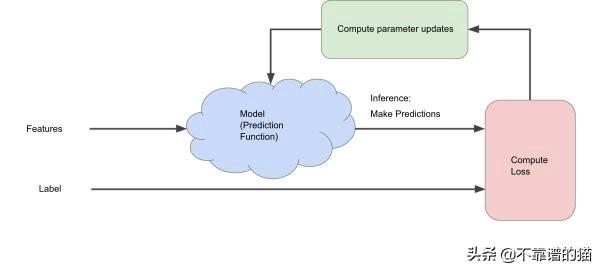批梯度下降法batch梯度下降针对每次梯度下降迭代处理所有训练实例。如果训练样本的数量很大，那么批梯度下降在计算上将非常昂贵。因此，如果训练实例的数量很大，则批梯度下降不是首选。相反，我们更喜欢使用随机梯度下降或mini-batch梯度下降。它是如何运作的？由于我们需要计算整个机器学习数据集上的梯度来执行一次更新，因此批梯度下降可能非常慢，并且对于内存中不适合的数据集来说很棘手。用任意值初始化参数(说θ1=θ2=…=θn= 0)后，我们使用以下关系式计算成本函数的梯度：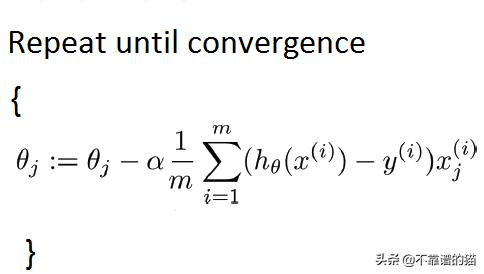其中“ m”是训练样本的数量。如果您有1万条记录，则需要将所有记录从磁盘读入内存。在计算了一次迭代的sigma之后，我们执行了一步。然后对每个步骤重复一次。这意味着收敛需要很长时间。因此，我们将倾向于使用其他方法。随机梯度下降法随机梯度下降中，每次迭代处理一个训练实例。因此，这比批梯度下降要快得多。但是，当训练样本的数量很大时，它也只处理一个样本，这可能会增加系统的开销，因为迭代的数量会非常大。它是如何工作的？算法的第一步是将整个训练集随机化。然后，对于每个参数的更新，我们在每次迭代中仅使用一个训练样本来计算成本函数的梯度。由于它在每次迭代中都使用一个训练样本，因此对于较大的机器学习数据集，此方法更快。用任意值初始化参数(说θ1=θ2=…=θn= 0)后，我们使用以下关系式计算成本函数的梯度：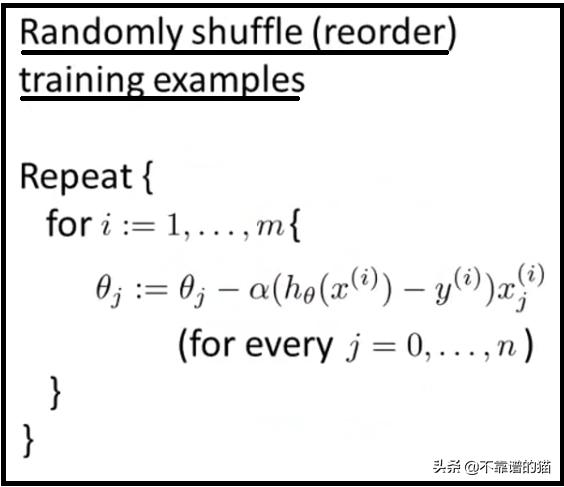其中“ m”是训练样本的数量选择第一个训练样本并使用该样本更新参数，然后选择第二个样本，依此类推然后选择第二个训练样本，并使用该样本更新参数，以此类推。选择第三个直到n。直到达到全局最小值。随机梯度下降法实际上不像批梯度下降法那样收敛，而是在接近全局最小值的区域内徘徊。Mini Batch梯度下降法Mini Batch梯度下降的速度比批梯度下降和随机梯度下降均快。在这里，每个迭代处理b个样本，其中b(m是训练样本的数量)。因此，即使训练样本数量很多，也要一次性处理b个训练样本的批次。因此，它适用于较大的训练样本，并且适用于较少的迭代次数。如何运作由于它是使用最广泛的算法，可以产生准确，快速的结果。减少了参数更新的方差，这可以导致更稳定的收敛。可以利用高度优化的矩阵，从而使梯度计算非常有效。用任意值初始化参数后，我们使用以下关系式计算成本函数的梯度：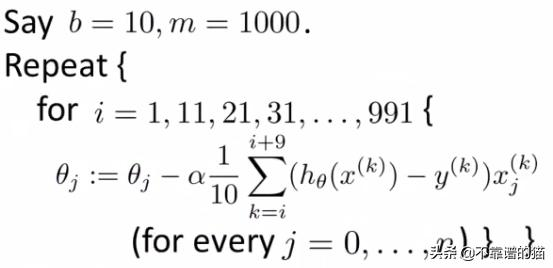alpha是学习率常见的Mini Batch大小在50到256之间，但是会因不同的应用而有所不同。最后批梯度下降，Mini Batch梯度下降和随机梯度下降之间的区别是用于执行一个更新步骤的样本数。经过微调的mini-batch，其性能优于梯度下降或随机梯度下降。基于准确度和时间消耗等参数的批量梯度下降法、小批量梯度下降法和随机梯度下降法的区别是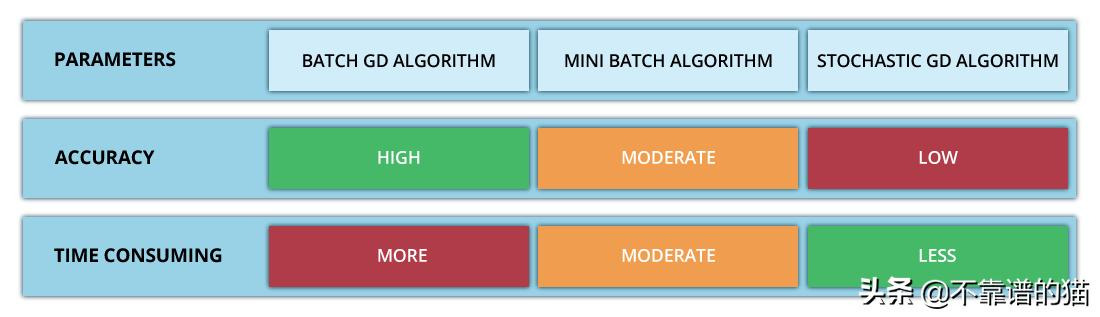展开全文• 本文转载于 https://www.jianshu.com/p/c7e642877b0e 这大概是我见过最好理解的一个版本 梯度下降的场景假设 梯度 梯度下降算法的数学解释 梯度下降算法实例 梯度下降算法的实现 Further reading ...机器学习经典算法
• 梯度下降算法最贴近生活的一个实例就是下山。假设我们在山上的某个位置，我们要如何走才能最快到达山底呢？答案很显然，沿着山腰最陡峭的方向向下走。具体方法步骤如下： 确定自己所在的地方 查看并找到四周最陡峭...
• 如有冒犯，还望谅解！ 梯度下降的场景假设 梯度 ...本文将从一个下山的场景开始，先提出梯度下降算法的基本思想，进而从数学上解释梯度下降算法的原理，最后实现一个简单的梯度下降算法实例！...函数优化
• 使用梯度下降算法进行学习（Learning with gradient descent） 1. 目标 我们希望有一个算法，能让我们找到权重和偏置，以至于网络的输出y(x) 能够拟合所有的训练输入x。 2. 代价函数（cost function） 定义一个...
• 梯度下降算法分类总结 引言 &nbsp; &nbsp;梯度下降法 (Gradient Descent Algorithm，GD) 是为目标函数J(θ)，如代价函数(cost function), 求解全局最小值（Global Minimum）的一种迭代算法。 为什么...
• 这三种算法都用于反向传播的优化损失函数算法。在每轮迭代中更新一次权重w，...1. 梯度下降算法： 主要优点：梯度更新准确 (1) 如果数据集比较小，完全可以采用全数据集(Full Batch Learning)的形式，采用全数据有...机器学习
• 1. 批量梯度下降算法： (1) 如果数据集比较小，完全可以采用全数据集(Full Batch Learning)的形式，采用全数据有两个好处： a.由全数据集确定的方向能够更好地代表样本总体，从而更准确地朝向极值所在的方向。b....
• 本文实例为大家分享了python批量梯度下降算法的具体代码，供大家参考，具体内容如下 问题： 将拥有两个自变量的二阶函数绘制到空间坐标系中，并通过批量梯度下降算法找到并绘制其极值点 大体思路： 首先，根据题意...
• 本文将从一个下山的场景开始，先提出梯度下降算法的基本思想，进而从数学上解释梯度下降算法的原理，解释为什么要用梯度，最后实现一个简单的梯度下降算法实例！ 2.梯度下降算法 2.1场景假设 梯度下降法的基本思想...梯度下降 机器学习 人工智能
• 在吴恩达的机器学习课程中，在介绍了批量梯度下降算法后，又介绍了随机梯度下降算法，随机梯度下降算法在处理大批量数据的时候运算速度优于批量梯度下降算法。 讲义中并未提及该算法的具体推导，仅列出了算法的流程...Matlab
• 神经网络优化方法-梯度下降算法 梯度下降算法主要用于优化单个参数的取值， 反向传播算法给出了一个高效的方式在所有参数上使用梯度下降算法。...通过以下是实例来解释梯度下降算法作用于损失函数的应用。 神..
• 采用动量梯度下降算法训练 BP 网络 采用贝叶斯正则化算法提高 BP 网络的推广能力 采用“提前停止”方法提高 BP 网络的推广能力bp神经网络
• 2.2 梯度下降算法 2.2.1 批量梯度下降算法 2.2.2 随机梯度下降算法 3.参考文献 1.前言 　这篇随笔,记录的是个人对于梯度算法的些许理解. 由于充斥着太多关于梯度算法的各种说明软文,所以呢? 本文是从一个...
• 梯度下降算法 引出案例 算法推导 数学实例 引出案例 目标：现已在山顶，要下山。 路径：一条平坦，一条陡峭。 结果：都能实现下山结果，平坦的路所用时间更长，陡峭的用时短。（假定该人速度相同） 下山最快...
• 梯度下降法是神经网络模型训练最常用的优化算法 对于深度学习，梯度下降法大部分的模型中都会遇到，其中也有不少学问 找到目标函数的梯度，而梯度代表的就是函数上升最快的方向 对于最小优化 哼～ ...神经网络 机器学习 人工智能 深度学习
• 本文将从一个下山的场景开始，先提出梯度下降算法的基本思想，进而从数学上解释梯度下降算法的原理，解释为什么要用梯度，最后实现一个简单的梯度下降算法实例！ 2.场景假设 梯度下降法的基本思想可以类比为一个......